# PCL Walkthrough

This tutorials will walk you through the components of your PCL installation, providing short descriptions of the modules, indicating where they are located and also listing the interaction between different components.

# Overview

PCL is split in a number of modular libraries. The most important set of released PCL modules is shown below:

 Filters Features Keypoints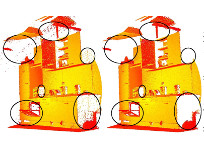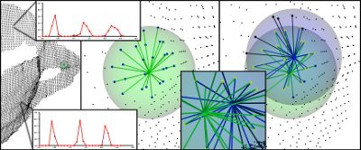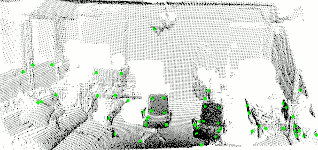Registration KdTree Octree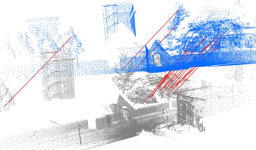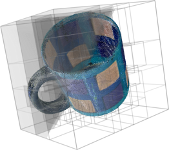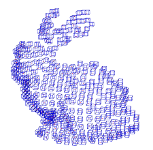Segmentation Sample Consensus Surface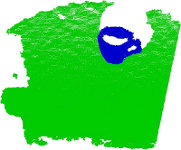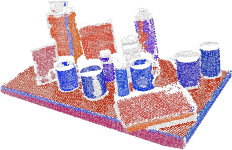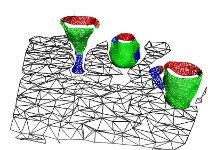Range Image I/O Visualization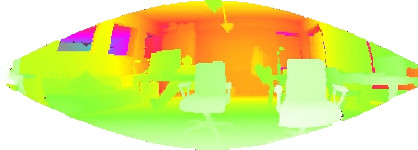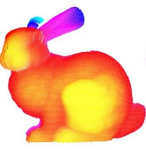Common Search# Filters

Background

An example of noise removal is presented in the figure below. Due to measurement errors, certain datasets present a large number of shadow points. This complicates the estimation of local point cloud 3D features. Some of these outliers can be filtered by performing a statistical analysis on each point’s neighborhood, and trimming those that do not meet a certain criteria. The sparse outlier removal implementation in PCL is based on the computation of the distribution of point to neighbor distances in the input dataset. For each point, the mean distance from it to all its neighbors is computed. By assuming that the resulting distribution is Gaussian with a mean and a standard deviation, all points whose mean distances are outside an interval defined by the global distances mean and standard deviation can be considered as outliers and trimmed from the dataset.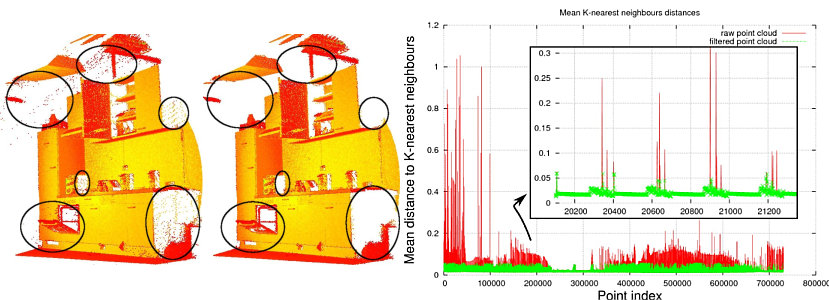Documentation: http://docs.pointclouds.org/trunk/group__filters.html

Interacts with:

Location:

• MAC OS X (Homebrew installation)
• Header files: $(PCL_PREFIX)/pcl-$(PCL_VERSION)/pcl/filters/
• Binaries: $(PCL_PREFIX)/bin/ • $(PCL_PREFIX) is the cmake installation prefix CMAKE_INSTALL_PREFIX, e.g., /usr/local/
• Linux
• Header files: $(PCL_PREFIX)/pcl-$(PCL_VERSION)/pcl/filters/
• Binaries: $(PCL_PREFIX)/bin/ • $(PCL_PREFIX) is the cmake installation prefix CMAKE_INSTALL_PREFIX, e.g., /usr/local/
• Windows
• Header files: $(PCL_DIRECTORY)/include/pcl-$(PCL_VERSION)/pcl/filters/
• Binaries: $(PCL_DIRECTORY)/bin/ • $(PCL_DIRECTORY) is the PCL installation directory, e.g., C:\Program Files\PCL $(PCL_VERSION)\ Top # Features Background A theoretical primer explaining how features work in PCL can be found in the 3D Features tutorial. The features library contains data structures and mechanisms for 3D feature estimation from point cloud data. 3D features are representations at certain 3D points, or positions, in space, which describe geometrical patterns based on the information available around the point. The data space selected around the query point is usually referred to as the k-neighborhood. The following figure shows a simple example of a selected query point, and its selected k-neighborhood.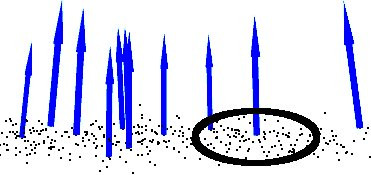An example of two of the most widely used geometric point features are the underlying surface’s estimated curvature and normal at a query point p. Both of them are considered local features, as they characterize a point using the information provided by its k closest point neighbors. For determining these neighbors efficiently, the input dataset is usually split into smaller chunks using spatial decomposition techniques such as octrees or kD-trees, and then closest point searches are performed in that space. Depending on the application one can opt for either determining a fixed number of k points in the vicinity of p, or all points which are found inside of a sphere of radius r centered at p. Unarguably, one the easiest methods for estimating the surface normals and curvature changes at a point p is to perform an eigendecomposition (i.e., compute the eigenvectors and eigenvalues) of the k-neighborhood point surface patch. Thus, the eigenvector corresponding to the smallest eigenvalue will approximate the surface normal n at point p, while the surface curvature change will be estimated from the eigenvalues aswith.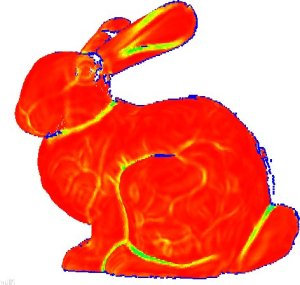Documentation: http://docs.pointclouds.org/trunk/group__features.html Interacts with: Location: • MAC OS X (Homebrew installation) • Header files: $(PCL_PREFIX)/pcl-$(PCL_VERSION)/pcl/features/ • Binaries: $(PCL_PREFIX)/bin/
• $(PCL_PREFIX) is the cmake installation prefix CMAKE_INSTALL_PREFIX, e.g., /usr/local/ • Linux • Header files: $(PCL_PREFIX)/pcl-$(PCL_VERSION)/pcl/filters/ • Binaries: $(PCL_PREFIX)/bin/
• $(PCL_PREFIX) is the cmake installation prefix CMAKE_INSTALL_PREFIX, e.g., /usr/local/ • Windows • Header files: $(PCL_DIRECTORY)/include/pcl-$(PCL_VERSION)/pcl/features/ • Binaries: $(PCL_DIRECTORY)/bin/
• $(PCL_DIRECTORY) is the PCL installation directory, e.g., C:\Program Files\PCL$(PCL_VERSION)\

Top

# Keypoints

Background

The keypoints library contains implementations of two point cloud keypoint detection algorithms. Keypoints (also referred to as interest points) are points in an image or point cloud that are stable, distinctive, and can be identified using a well-defined detection criterion. Typically, the number of interest points in a point cloud will be much smaller than the total number of points in the cloud, and when used in combination with local feature descriptors at each keypoint, the keypoints and descriptors can be used to form a compact—yet descriptive—representation of the original data.

The figure below shows the output of NARF keypoints extraction from a range image: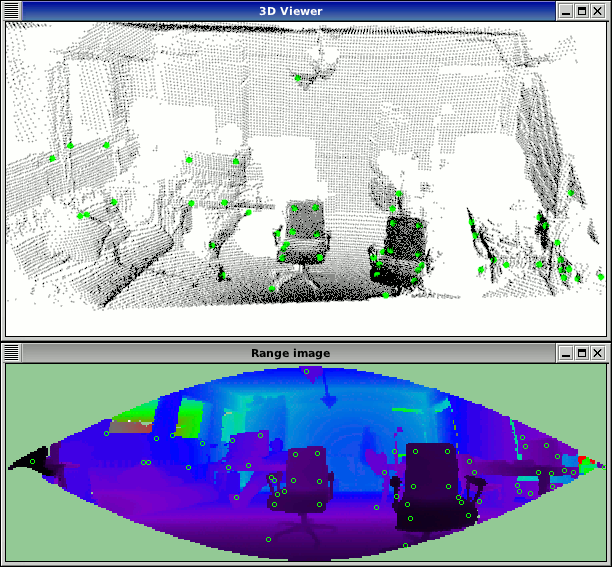Documentation: http://docs.pointclouds.org/trunk/group__keypoints.html

Interacts with:

Location:

• MAC OS X (Homebrew installation)
• Header files: $(PCL_PREFIX)/pcl-$(PCL_VERSION)/pcl/keypoints/
• Binaries: $(PCL_PREFIX)/bin/ • $(PCL_PREFIX) is the cmake installation prefix CMAKE_INSTALL_PREFIX, e.g., /usr/local/
• Linux
• Header files: $(PCL_PREFIX)/pcl-$(PCL_VERSION)/pcl/filters/
• Binaries: $(PCL_PREFIX)/bin/ • $(PCL_PREFIX) is the cmake installation prefix CMAKE_INSTALL_PREFIX, e.g., /usr/local/
• Windows
• Header files: $(PCL_DIRECTORY)/include/pcl-$(PCL_VERSION)/pcl/keypoints/
• Binaries: $(PCL_DIRECTORY)/bin/ • $(PCL_DIRECTORY) is the PCL installation directory, e.g., C:\Program Files\PCL (PCL_VERSION)\ Top # Registration Background Combining several datasets into a global consistent model is usually performed using a technique called registration. The key idea is to identify corresponding points between the data sets and find a transformation that minimizes the distance (alignment error) between corresponding points. This process is repeated, since correspondence search is affected by the relative position and orientation of the data sets. Once the alignment errors fall below a given threshold, the registration is said to be complete. The registration library implements a plethora of point cloud registration algorithms for both organized and unorganized (general purpose) datasets. For instance, PCL contains a set of powerful algorithms that allow the estimation of multiple sets of correspondences, as well as methods for rejecting bad correspondences, and estimating transformations in a robust manner.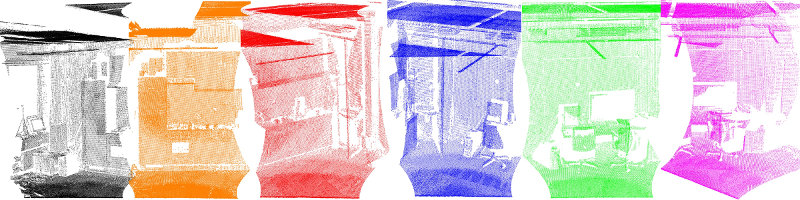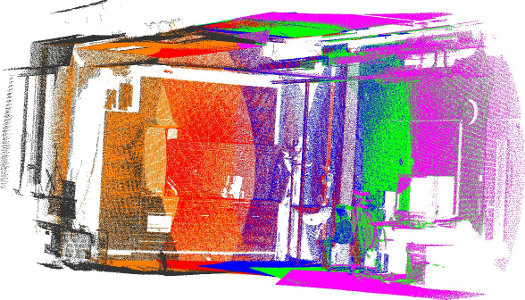Interacts with: Location: • MAC OS X (Homebrew installation) • Header files: (PCL_PREFIX)/pcl-$(PCL_VERSION)/pcl/registration/ • Binaries: $(PCL_PREFIX)/bin/
• $(PCL_PREFIX) is the cmake installation prefix CMAKE_INSTALL_PREFIX, e.g., /usr/local/ • Linux • Header files: $(PCL_PREFIX)/pcl-$(PCL_VERSION)/pcl/filters/ • Binaries: $(PCL_PREFIX)/bin/
• $(PCL_PREFIX) is the cmake installation prefix CMAKE_INSTALL_PREFIX, e.g., /usr/local/ • Windows • Header files: $(PCL_DIRECTORY)/include/pcl-$(PCL_VERSION)/pcl/registration/ • Binaries: $(PCL_DIRECTORY)/bin/
• $(PCL_DIRECTORY) is the PCL installation directory, e.g., C:\Program Files\PCL$(PCL_VERSION)\

Top

# Kd-tree

Background

A theoretical primer explaining how Kd-trees work can be found in the Kd-tree tutorial.

The kdtree library provides the kd-tree data-structure, using FLANN, that allows for fast nearest neighbor searches.

A Kd-tree (k-dimensional tree) is a space-partitioning data structure that stores a set of k-dimensional points in a tree structure that enables efficient range searches and nearest neighbor searches. Nearest neighbor searches are a core operation when working with point cloud data and can be used to find correspondences between groups of points or feature descriptors or to define the local neighborhood around a point or points.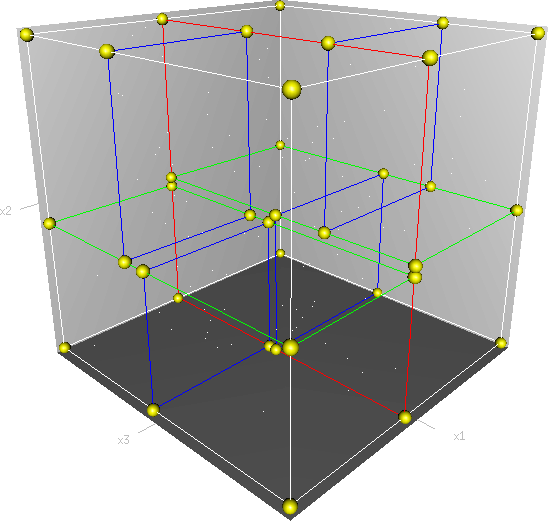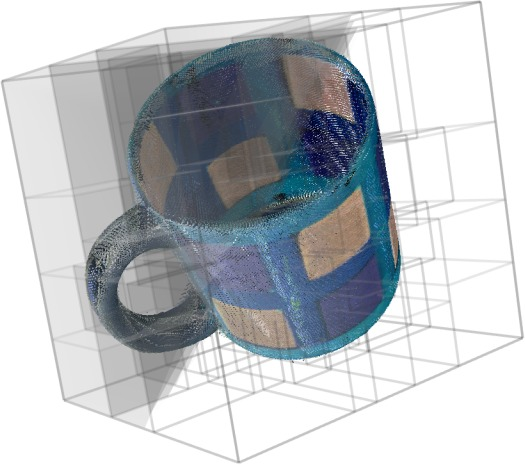Documentation: http://docs.pointclouds.org/trunk/group__kdtree.html

Interacts with: Common

Location:

• MAC OS X (Homebrew installation)
• Header files: $(PCL_PREFIX)/pcl-$(PCL_VERSION)/pcl/kdtree/
• Binaries: $(PCL_PREFIX)/bin/ • $(PCL_PREFIX) is the cmake installation prefix CMAKE_INSTALL_PREFIX, e.g., /usr/local/
• Linux
• Header files: $(PCL_PREFIX)/pcl-$(PCL_VERSION)/pcl/filters/
• Binaries: $(PCL_PREFIX)/bin/ • $(PCL_PREFIX) is the cmake installation prefix CMAKE_INSTALL_PREFIX, e.g., /usr/local/
• Windows
• Header files: $(PCL_DIRECTORY)/include/pcl-$(PCL_VERSION)/pcl/kdtree/
• Binaries: $(PCL_DIRECTORY)/bin/ • $(PCL_DIRECTORY) is the PCL installation directory, e.g., C:\Program Files\PCL $(PCL_VERSION)\ Top # Octree Background The octree library provides efficient methods for creating a hierarchical tree data structure from point cloud data. This enables spatial partitioning, downsampling and search operations on the point data set. Each octree node has either eight children or no children. The root node describes a cubic bounding box which encapsulates all points. At every tree level, this space becomes subdivided by a factor of 2 which results in an increased voxel resolution. The octree implementation provides efficient nearest neighbor search routines, such as “Neighbors within Voxel Search”, “K Nearest Neighbor Search” and “Neighbors within Radius Search”. It automatically adjusts its dimension to the point data set. A set of leaf node classes provide additional functionality, such as spacial “occupancy” and “point density per voxel” checks. Functions for serialization and deserialization enable to efficiently encode the octree structure into a binary format. Furthermore, a memory pool implementation reduces expensive memory allocation and deallocation operations in scenarios where octrees needs to be created at high rate. The following figure illustrates the voxel bounding boxes of an octree nodes at lowest tree level. The octree voxels are surrounding every 3D point from the Stanford bunny’s surface. The red dots represent the point data. This image is created with the octree_viewer.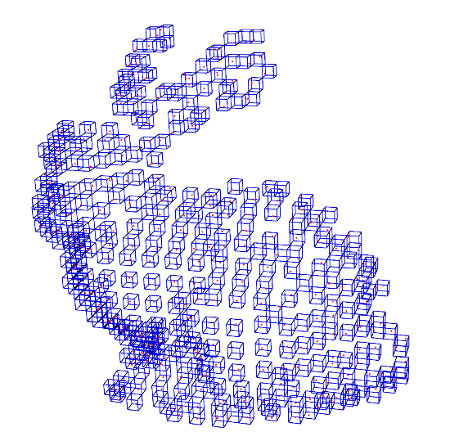Documentation: http://docs.pointclouds.org/trunk/group__octree.html Interacts with: Common Location: • MAC OS X (Homebrew installation) • Header files: $(PCL_PREFIX)/pcl-$(PCL_VERSION)/pcl/octree/ • Binaries: $(PCL_PREFIX)/bin/
• $(PCL_PREFIX) is the cmake installation prefix CMAKE_INSTALL_PREFIX, e.g., /usr/local/ • Linux • Header files: $(PCL_PREFIX)/pcl-$(PCL_VERSION)/pcl/filters/ • Binaries: $(PCL_PREFIX)/bin/
• $(PCL_PREFIX) is the cmake installation prefix CMAKE_INSTALL_PREFIX, e.g., /usr/local/ • Windows • Header files: $(PCL_DIRECTORY)/include/pcl-$(PCL_VERSION)/pcl/octree/ • Binaries: $(PCL_DIRECTORY)/bin/
• $(PCL_DIRECTORY) is the PCL installation directory, e.g., C:\Program Files\PCL$(PCL_VERSION)\

Top

# Segmentation

Background

The segmentation library contains algorithms for segmenting a point cloud into distinct clusters. These algorithms are best suited for processing a point cloud that is composed of a number of spatially isolated regions. In such cases, clustering is often used to break the cloud down into its constituent parts, which can then be processed independently.

A theoretical primer explaining how clustering methods work can be found in the cluster extraction tutorial. The two figures illustrate the results of plane model segmentation (left) and cylinder model segmentation (right).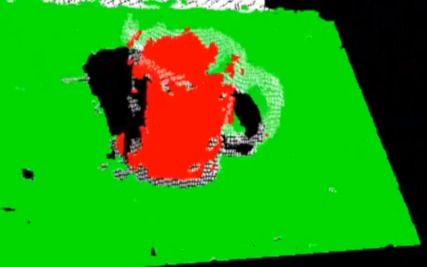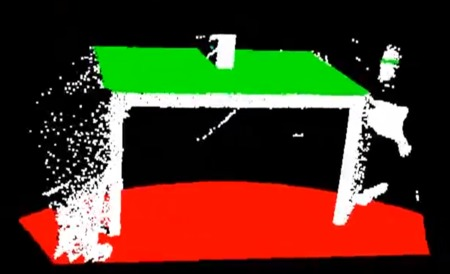Interacts with:

Location:

• MAC OS X (Homebrew installation)
• Header files: $(PCL_PREFIX)/pcl-$(PCL_VERSION)/pcl/segmentation/
• Binaries: $(PCL_PREFIX)/bin/ • $(PCL_PREFIX) is the cmake installation prefix CMAKE_INSTALL_PREFIX, e.g., /usr/local/
• Linux
• Header files: $(PCL_PREFIX)/pcl-$(PCL_VERSION)/pcl/filters/
• Binaries: $(PCL_PREFIX)/bin/ • $(PCL_PREFIX) is the cmake installation prefix CMAKE_INSTALL_PREFIX, e.g., /usr/local/
• Windows
• Header files: $(PCL_DIRECTORY)/include/pcl-$(PCL_VERSION)/pcl/segmentation/
• Binaries: $(PCL_DIRECTORY)/bin/ • $(PCL_DIRECTORY) is the PCL installation directory, e.g., C:\Program Files\PCL $(PCL_VERSION)\ Top # Sample Consensus Background The sample_consensus library holds SAmple Consensus (SAC) methods like RANSAC and models like planes and cylinders. These can combined freely in order to detect specific models and their parameters in point clouds. A theoretical primer explaining how sample consensus algorithms work can be found in the Random Sample Consensus tutorial Some of the models implemented in this library include: lines, planes, cylinders, and spheres. Plane fitting is often applied to the task of detecting common indoor surfaces, such as walls, floors, and table tops. Other models can be used to detect and segment objects with common geometric structures (e.g., fitting a cylinder model to a mug).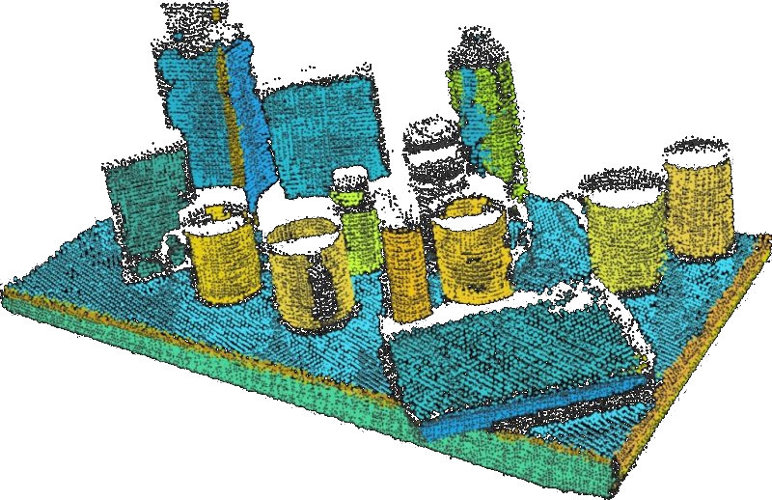Interacts with: Common Location: • MAC OS X (Homebrew installation) • Header files: $(PCL_PREFIX)/pcl-$(PCL_VERSION)/pcl/sample_consensus/ • Binaries: $(PCL_PREFIX)/bin/
• $(PCL_PREFIX) is the cmake installation prefix CMAKE_INSTALL_PREFIX, e.g., /usr/local/ • Linux • Header files: $(PCL_PREFIX)/pcl-$(PCL_VERSION)/pcl/filters/ • Binaries: $(PCL_PREFIX)/bin/
• $(PCL_PREFIX) is the cmake installation prefix CMAKE_INSTALL_PREFIX, e.g., /usr/local/ • Windows • Header files: $(PCL_DIRECTORY)/include/pcl-$(PCL_VERSION)/pcl/sample_consensus/ • Binaries: $(PCL_DIRECTORY)/bin/
• $(PCL_DIRECTORY) is the PCL installation directory, e.g., C:\Program Files\PCL$(PCL_VERSION)\

Top

# Surface

Background

The surface library deals with reconstructing the original surfaces from 3D scans. Depending on the task at hand, this can be for example the hull, a mesh representation or a smoothed/resampled surface with normals.

Smoothing and resampling can be important if the cloud is noisy, or if it is composed of multiple scans that are not aligned perfectly. The complexity of the surface estimation can be adjusted, and normals can be estimated in the same step if needed.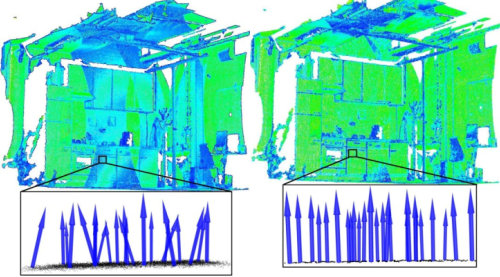Meshing is a general way to create a surface out of points, and currently there are two algorithms provided: a very fast triangulation of the original points, and a slower meshing that does smoothing and hole filling as well.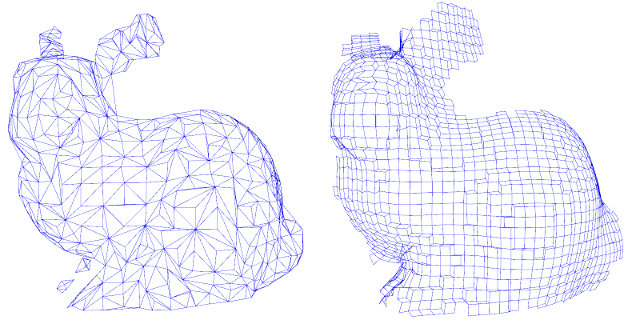Creating a convex or concave hull is useful for example when there is a need for a simplified surface representation or when boundaries need to be extracted.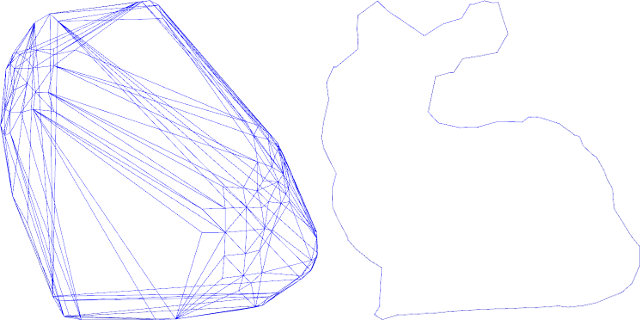Documentation: http://docs.pointclouds.org/trunk/group__surface.html

Interacts with:

Location:

• MAC OS X (Homebrew installation)
• Header files: $(PCL_PREFIX)/pcl-$(PCL_VERSION)/pcl/surface/
• Binaries: $(PCL_PREFIX)/bin/ • $(PCL_PREFIX) is the cmake installation prefix CMAKE_INSTALL_PREFIX, e.g., /usr/local/
• Linux
• Header files: $(PCL_PREFIX)/pcl-$(PCL_VERSION)/pcl/filters/
• Binaries: $(PCL_PREFIX)/bin/ • $(PCL_PREFIX) is the cmake installation prefix CMAKE_INSTALL_PREFIX, e.g., /usr/local/
• Windows
• Header files: $(PCL_DIRECTORY)/include/pcl-$(PCL_VERSION)/pcl/surface/
• Binaries: $(PCL_DIRECTORY)/bin/ • $(PCL_DIRECTORY) is the PCL installation directory, e.g., C:\Program Files\PCL $(PCL_VERSION)\ Top # Range Image Background The range_image library contains two classes for representing and working with range images. A range image (or depth map) is an image whose pixel values represent a distance or depth from the sensor’s origin. Range images are a common 3D representation and are often generated by stereo or time-of-flight cameras. With knowledge of the camera’s intrinsic calibration parameters, a range image can be converted into a point cloud. Note: range_image is now a part of Common module.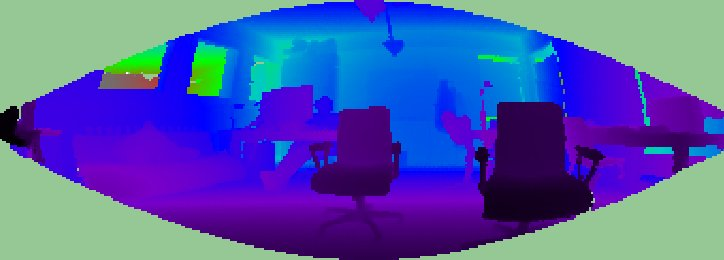Interacts with: Common Location: • MAC OS X (Homebrew installation) • Header files: $(PCL_PREFIX)/pcl-$(PCL_VERSION)/pcl/range_image/ • Binaries: $(PCL_PREFIX)/bin/
• $(PCL_PREFIX) is the cmake installation prefix CMAKE_INSTALL_PREFIX, e.g., /usr/local/ • Linux • Header files: $(PCL_PREFIX)/pcl-$(PCL_VERSION)/pcl/filters/ • Binaries: $(PCL_PREFIX)/bin/
• $(PCL_PREFIX) is the cmake installation prefix CMAKE_INSTALL_PREFIX, e.g., /usr/local/ • Windows • Header files: $(PCL_DIRECTORY)/include/pcl-$(PCL_VERSION)/pcl/range_image/ • Binaries: $(PCL_DIRECTORY)/bin/
• $(PCL_DIRECTORY) is the PCL installation directory, e.g., C:\Program Files\PCL$(PCL_VERSION)\

Top

# I/O

Background

The io library contains classes and functions for reading and writing point cloud data (PCD) files, as well as capturing point clouds from a variety of sensing devices. An introduction to some of these capabilities can be found in the following tutorials:

Documentation: http://docs.pointclouds.org/trunk/group__io.html

Interacts with:

Location:

• MAC OS X (Homebrew installation)
• Header files: $(PCL_PREFIX)/pcl-$(PCL_VERSION)/pcl/io/
• Binaries: $(PCL_PREFIX)/bin/ • $(PCL_PREFIX) is the cmake installation prefix CMAKE_INSTALL_PREFIX, e.g., /usr/local/
• Linux
• Header files: $(PCL_PREFIX)/pcl-$(PCL_VERSION)/pcl/filters/
• Binaries: $(PCL_PREFIX)/bin/ • $(PCL_PREFIX) is the cmake installation prefix CMAKE_INSTALL_PREFIX, e.g., /usr/local/
• Windows
• Header files: $(PCL_DIRECTORY)/include/pcl-$(PCL_VERSION)/pcl/io/
• Binaries: $(PCL_DIRECTORY)/bin/ • $(PCL_DIRECTORY) is the PCL installation directory, e.g., C:\Program Files\PCL $(PCL_VERSION)\ Top # Visualization Background The visualization library was built for the purpose of being able to quickly prototype and visualize the results of algorithms operating on 3D point cloud data. Similar to OpenCV’s highgui routines for displaying 2D images and for drawing basic 2D shapes on screen, the library offers: methods for rendering and setting visual properties (colors, point sizes, opacity, etc) for any n-D point cloud datasets in pcl::PointCloud<T> format;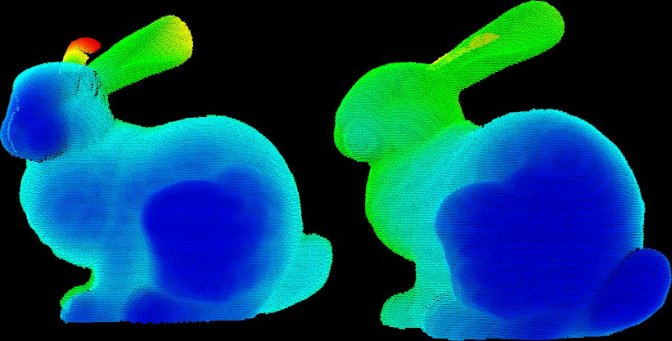methods for drawing basic 3D shapes on screen (e.g., cylinders, spheres,lines, polygons, etc) either from sets of points or from parametric equations;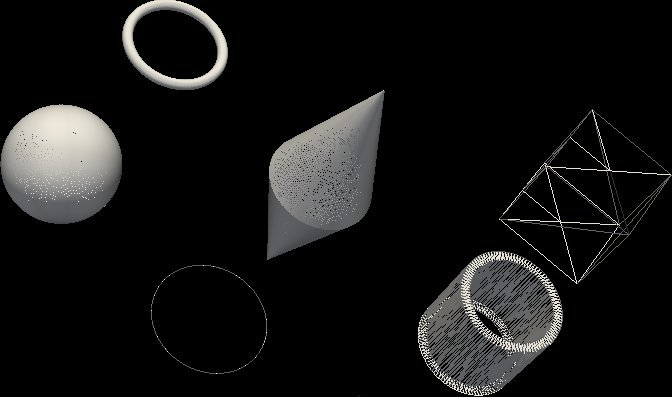a histogram visualization module (PCLHistogramVisualizer) for 2D plots;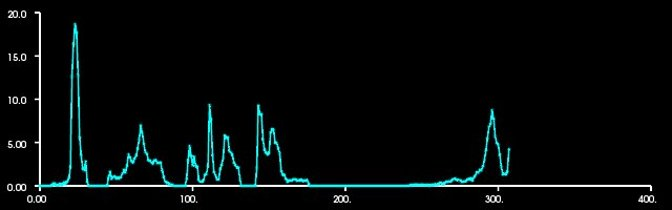a multitude of Geometry and Color handlers for pcl::PointCloud<T> datasets;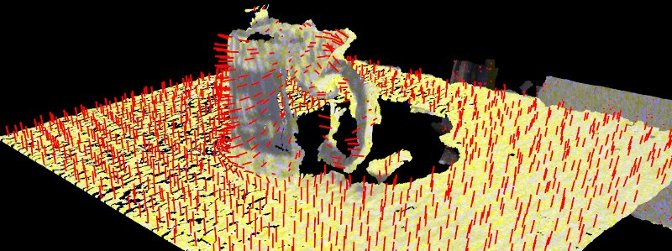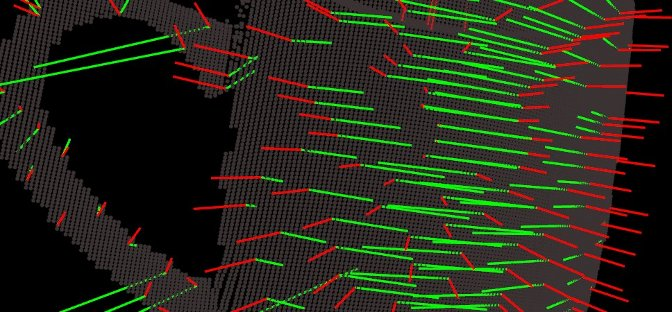a pcl::RangeImage visualization module.The package makes use of the VTK library for 3D rendering for range image and 2D operations. For implementing your own visualizers, take a look at the tests and examples accompanying the library. Interacts with: Location: • MAC OS X (Homebrew installation) • Header files: $(PCL_PREFIX)/pcl-$(PCL_VERSION)/pcl/visualization/ • Binaries: $(PCL_PREFIX)/bin/
• $(PCL_PREFIX) is the cmake installation prefix CMAKE_INSTALL_PREFIX, e.g., /usr/local/ • Linux • Header files: $(PCL_PREFIX)/pcl-$(PCL_VERSION)/pcl/filters/ • Binaries: $(PCL_PREFIX)/bin/
• $(PCL_PREFIX) is the cmake installation prefix CMAKE_INSTALL_PREFIX, e.g., /usr/local/ • Windows • Header files: $(PCL_DIRECTORY)/include/pcl-$(PCL_VERSION)/pcl/visualization/ • Binaries: $(PCL_DIRECTORY)/bin/
• $(PCL_DIRECTORY) is the PCL installation directory, e.g., C:\Program Files\PCL$(PCL_VERSION)\

Top

# Common

Background

The common library contains the common data structures and methods used by the majority of PCL libraries. The core data structures include the PointCloud class and a multitude of point types that are used to represent points, surface normals, RGB color values, feature descriptors, etc. It also contains numerous functions for computing distances/norms, means and covariances, angular conversions, geometric transformations, and more.

Location:

• MAC OS X (Homebrew installation)
• Header files: $(PCL_PREFIX)/pcl-$(PCL_VERSION)/pcl/common/
• Binaries: $(PCL_PREFIX)/bin/ • $(PCL_PREFIX) is the cmake installation prefix CMAKE_INSTALL_PREFIX, e.g., /usr/local/
• Linux
• Header files: $(PCL_PREFIX)/pcl-$(PCL_VERSION)/pcl/common/
• Binaries: $(PCL_PREFIX)/bin/ • $(PCL_PREFIX) is the cmake installation prefix CMAKE_INSTALL_PREFIX, e.g., /usr/local/
• Windows
• Header files: $(PCL_DIRECTORY)/include/pcl-$(PCL_VERSION)/pcl/common/
• Binaries: $(PCL_DIRECTORY)/bin/ • $(PCL_DIRECTORY) is the PCL installation directory, e.g., C:\Program Files\PCL \$(PCL_VERSION)\

Top

# Binaries

This section provides a quick reference for some of the common tools in PCL.

• pcl_viewer: a quick way for visualizing PCD (Point Cloud Data) files. More information about PCD files can be found in the PCD file format tutorial.

Syntax is: pcl_viewer <file_name 1..N>.<pcd or vtk> <options>, where options are:

-bc r,g,b = background color

-fc r,g,b = foreground color

-ps X = point size (1..64)

-opaque X = rendered point cloud opacity (0..1)

-ax n = enable on-screen display of XYZ axes and scale them to n

-ax_pos X,Y,Z = if axes are enabled, set their X,Y,Z position in space (default 0,0,0)

-cam (*) = use given camera settings as initial view

(*) [Clipping Range / Focal Point / Position / ViewUp / Distance / Field of View Y / Window Size / Window Pos] or use a <filename.cam> that contains the same information.

-multiview 0/1 = enable/disable auto-multi viewport rendering (default disabled)

-normals 0/X = disable/enable the display of every Xth point’s surface normal as lines (default disabled) -normals_scale X = resize the normal unit vector size to X (default 0.02)

-pc 0/X = disable/enable the display of every Xth point’s principal curvatures as lines (default disabled) -pc_scale X = resize the principal curvatures vectors size to X (default 0.02)

(Note: for multiple .pcd files, provide multiple -{fc,ps,opaque} parameters; they will be automatically assigned to the right file)

Usage example:

pcl_viewer -multiview 1 data/partial_cup_model.pcd data/partial_cup_model.pcd data/partial_cup_model.pcd

The above will load the partial_cup_model.pcd file 3 times, and will create a multi-viewport rendering (-multiview 1).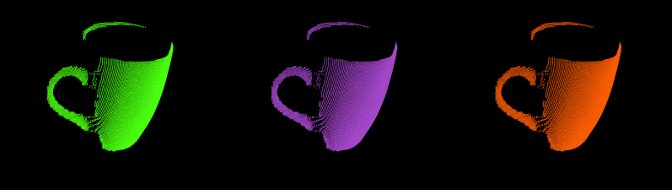• pcd_convert_NaN_nan: converts “NaN” values to “nan” values. (Note: Starting with PCL version 1.0.1 the string representation for NaN is “nan”.)

Usage example:

pcd_convert_NaN_nan input.pcd output.pcd

• convert_pcd_ascii_binary: converts PCD (Point Cloud Data) files from ASCII to binary and viceversa.

Usage example:

convert_pcd_ascii_binary <file_in.pcd> <file_out.pcd> 0/1/2 (ascii/binary/binary_compressed) [precision (ASCII)]

• concatenate_points_pcd: concatenates the points of two or more PCD (Point Cloud Data) files into a single PCD file.

Usage example:

concatenate_points_pcd <filename 1..N.pcd>

(Note: the resulting PCD file will be output.pcd)

• pcd2vtk: converts PCD (Point Cloud Data) files to the VTK format.

Usage example:

pcd2vtk input.pcd output.vtk

• pcd2ply: converts PCD (Point Cloud Data) files to the PLY format.

Usage example:

pcd2ply input.pcd output.ply

• mesh2pcd: convert a CAD model to a PCD (Point Cloud Data) file, using ray tracing operations.

Syntax is: mesh2pcd input.{ply,obj} output.pcd <options>, where options are:

-level X = tessellated sphere level (default: 2)

-resolution X = the sphere resolution in angle increments (default: 100 deg)

-leaf_size X = the XYZ leaf size for the VoxelGrid – for data reduction (default: 0.010000 m)

• octree_viewer: allows the visualization of octrees

Syntax is: octree_viewer <file_name.pcd> <octree resolution>

Usage example:

Example: ./octree_viewer ../../test/bunny.pcd 0.02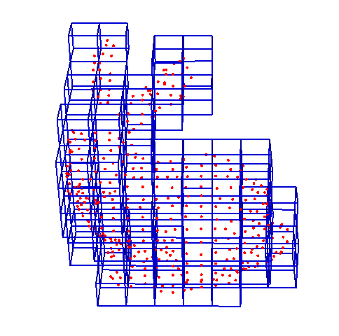Top